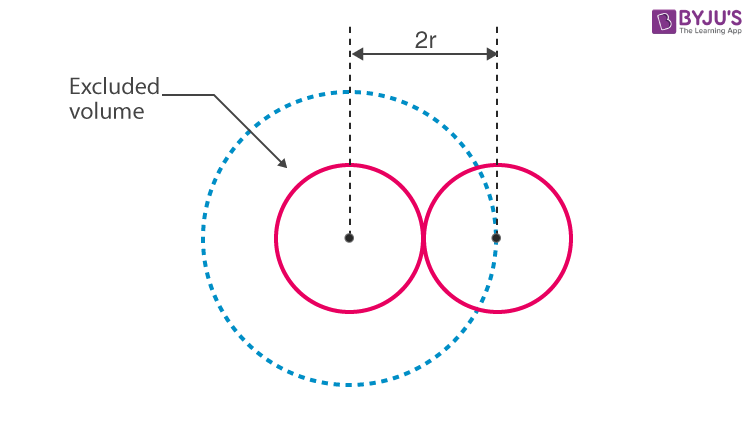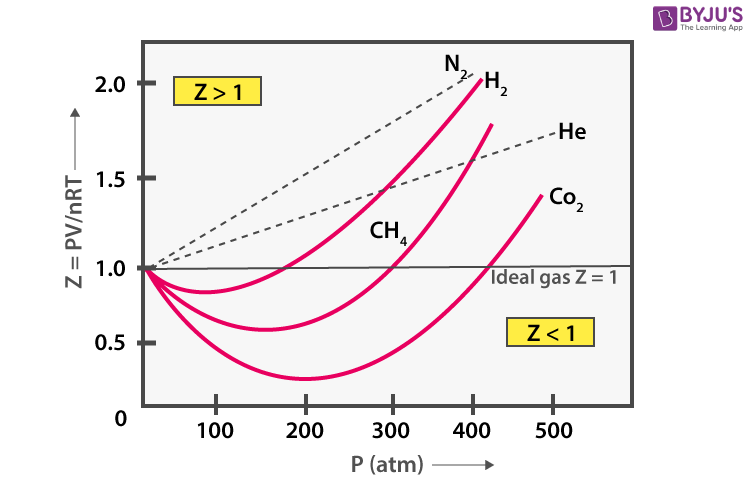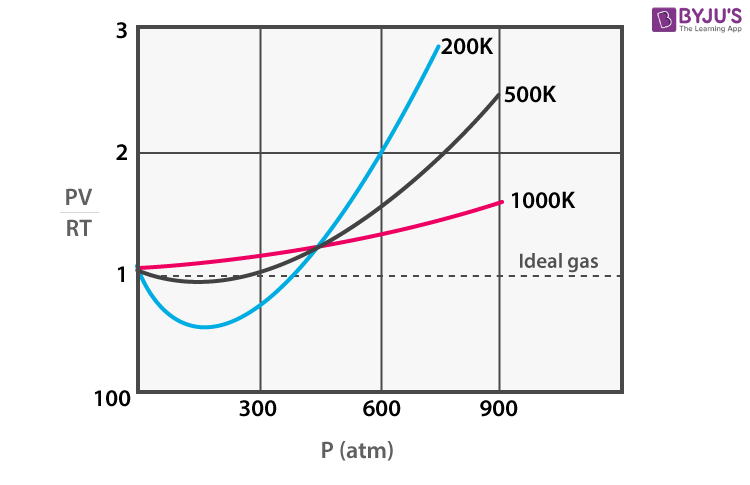Win up to 100% scholarship on Aakash BYJU'S JEE/NEET courses with ABNAT Win up to 100% scholarship on Aakash BYJU'S JEE/NEET courses with ABNAT

# Van der Waals Equation

The Van der Waals equation was derived by Johannes Diderik van der Waals in the year 1873. The equation is basically a modified version of the Ideal Gas Law which states that gases consist of point masses that undergo perfectly elastic collisions. However, this law fails to explain the behaviour of real gases. Therefore, the Van der Waals equation was devised, and it helps us define the physical state of a real gas.

More significantly, the Van der Waals equation takes into consideration the molecular size and molecular interaction forces (attractive and repulsive forces). Sometimes, it is also referred to as the Van der Waals equation of state.

## What Is Van der Waals Equation?

The Van der Waals equation is an equation relating the relationship between the pressure, volume, temperature, and amount of real gases. For a real gas containing ‘n’ moles, the equation is written as;

$$\begin{array}{l}\left( P+\frac{a{{n}^{2}}}{{{V}^{2}}} \right)\left( V-nb \right)=n\,R\,T\,\end{array}$$

Where P, V, T, and n are the pressure, volume, temperature and moles of the gas, respectively, and ‘a’ and ‘b’ constants specific to each gas.

The equation can further be written as:

1.  Cube power of volume:
$$\begin{array}{l}{{V}^{3}}-\left( b+\frac{RT}{P} \right){{V}^{2}}+\frac{a}{P}V-\frac{ab}{P}=0\end{array}$$
2. Reduced equation (Law of corresponding states) in terms of critical constants:
$$\begin{array}{l}\left( \pi +\frac{3}{{{\varphi }^{2}}} \right)\left( 3\varphi -1 \right)=8\tau :\,\,where\,\,\pi =\frac{P}{Pc},\varphi =\frac{V}{Vc}\,\,\,and\,\,\tau =\frac{T}{Tc}\end{array}$$

### Units of Van der Waals Constants

• Unit of “a” and is atm lit² mol⁻²
• Unit of “b” litre mol⁻¹

## Van der Waals Equation Derivation

Van der Waals equation derivation is based on correcting the pressure and volume of the ideal gases given by the Kinetic Theory of Gases. Another derivation is also used, which is based on the potentials of the particles.  Nonetheless, both derivations help us establish the same relationship.

### Van der Waals Equation of State for Real Gases – Derivation

The kinetic theory of ideal gases assumes the gaseous particles as –

• Point masses without any volume
• Independent having no interactions
• Undergo perfectly elastic collisions

In practice, Van der Waals assumed that gaseous particles –

1. Are hard spheres.
2. Have definite volume, and hence cannot be compressed beyond a limit.
3. Two particles at close range interact and have an exclusive spherical volume around them.

#### Volume Correction in Van der Waals Equation

As the particles have a definite volume, the volume available for their movement is not the entire container volume but less.

Volume in the ideal gas is hence an overestimation and has to be reduced for real gases.

The volume of the real gas VR = Volume of the container/ideal gas (VI) – Correction factor(b)

VR = VI – b.

If the particles are independent, then,

Total volume of the particle = number of particle x volume of one particle

$$\begin{array}{l}=\left( n\frac{4}{3}\pi {{r}^{3}} \right)\end{array}$$

But, the particles are not independent, and they do interact.

Van der Waals considered two hard-sphere particles can come as close as to touch each other, and they will not allow any other particle to enter into that volume, as shown in the diagram.The two-sphere model has a total radius of ‘2r’ (r is the radius of the sphere particle) and volume of

$$\begin{array}{l}\frac{4}{3}\pi 2{{r}^{3}}=8\times \frac{4}{3}\pi {{r}^{3}}=8\times\end{array}$$
volume of a single particle.

Then, each of the two particles has a sphere of influence of 4 times the volume of the particle.

Volume correction for each particle is not the volume of the particle but four times of it

$$\begin{array}{l}=b=4\times \frac{4}{3}\pi {{r}^{3}}\end{array}$$

Volume correction for ‘n’ particles

$$\begin{array}{l}=nb=4n\times \frac{4}{3}\pi {{r}^{3}}\end{array}$$

Volume (V) of the real gas = Vi – nb

Also Read: Van der Waals Forces

#### Pressure Correction in Van der Waals Equation

Gaseous particles do interact. For inside particles, the interactions cancel each other. But, the particles on the surface and near the walls of the container do not have particles above the surface and on the walls. So, there will be net interactions or pulling of the bulk molecules towards the bulk that is away from the walls and surface. The molecules experiencing a net interaction away from the walls will hit the walls with less force and pressure. Hence, in real gases, the particles exhibit lower pressure than shown by ideal gases.

The reduction in pressure α square of the particle density in bulk α (particle density/volume)2

$$\begin{array}{l}\alpha \frac{{{n}^{2}}}{{{V}^{2}}}=a\frac{{{n}^{2}}}{{{V}^{2}}};\end{array}$$
where, a is the proportionality constant.

Pressure of the real gas,

$$\begin{array}{l}Pi=\Pr +a\frac{{{n}^{2}}}{{{V}^{2}}}\end{array}$$

Substituting the pressure and volume correction in the ideal gas equation, we get the Van der Waals equation for real gases as:

$$\begin{array}{l}\left( \Pr +a\frac{{{n}^{2}}}{{{V}^{2}}} \right)\left( Vi-nb \right)=nRT\end{array}$$
or in general,
$$\begin{array}{l}\left({P}+a\frac{{{n}^{2}}}{{{V}^{2}}} \right)\left( V-nb \right)=nRT\end{array}$$

Here, ‘a’ and ‘b’ are Van der Waals constants and contain positive values. The constants are characteristic of the individual gas. When gas is ideal, or it behaves ideally, then both the constants will be zero. Generally, a constant helps in the correction of the intermolecular forces, while the b constant helps in making adjustments for the volume occupied by the gas particles.

## Ideal Gas Equation and Van der Waals Equation

Ideal gas equation is given as PV = nRT

Van der Waals equation is

$$\begin{array}{l}\left({P}+a\frac{{{n}^{2}}}{{{V}^{2}}} \right)(V -nb) = nRT\end{array}$$

At constant temperature, a decrease in pressure increases the volume (V). Hence at low pressures, the volume will be larger. So, the correction factor in pressure

$$\begin{array}{l}\left( a\frac{{{n}^{2}}}{{{V}^{2}}} \right)\end{array}$$
becomes very small and negligible.

Again, the volume of the gas will be larger compared to the volume of the molecules (n, b). Hence, the volume correction also will be small and negligible.

As the correction factor becomes negligible, the pressure and volume of the real gases will be equal to that of ideal gases. Interestingly, all real gases behave like ideal gases at low pressures and high temperatures.

### Merits of Van der Waals Equation of State

1. Able to predict the behaviour of gases better than the ideal gas equation
2. Applicable not only to gases but to all fluids
3. The arrangement of the equation in a cubic equation in volume. The cubic equation gives three volumes that are useful for calculating the volume at and below critical temperatures.
$$\begin{array}{l}{{V}^{3}}-\left( b+\frac{RT}{P} \right){{V}^{2}}+\frac{a}{P}V-\frac{ab}{P}=0\end{array}$$
4. Able to calculate the critical conditions of liquefaction and derive an expression of the Principle of Corresponding States.
$$\begin{array}{l}\left( \pi +\frac{3}{{{\varphi }^{2}}} \right)\left( 3\varphi -1 \right)=8\tau :\,\,where,\,\,\pi =\frac{P}{Pc},\varphi =\frac{V}{Vc}\,\,and\,\,\tau =\frac{T}{Tc}\end{array}$$

### Demerits of Van der Waals Equation

1. The equation gives more accurate results for all real gases only above the critical temperature.
2. The results are acceptable below the critical temperature.
3. The equation completely fails in the transition phase of gas to the liquid below a critical temperature.

In any case, Van der Waals theory helps us to develop an approximation for real gases at high pressures and also predict the behaviour of non-ideal gases.

## Solving Van der Waals Equation Problems

1.  At 250 K, the activation energy for a gas-phase reaction was determined to be 6.500 kJ mol-1. What percentage of gaseous molecules would be expected to have less than this energy at 250 K?

The expression in terms of moles for the distribution of molecular energies, nE = ne-E/RT, the fraction of the total moles, (n), that have energy E or greater, (nE), as = nE/n = e-E/RT

At T = 250 K and for E = 6.500 kJ mol-1 = 6500 J mol-1, so, nE/n = e-6500/(8.314 x 250) = 0.044 or 4.4%.

Therefore, the percentage of molecules that have less than 6.500 kJ mol-1 energy = 100.0 – 4.4 = 95.6%.

2. Choose the correct option.

a) Increasing temperature increases the distribution of molecular velocities.

b) Larger the mass lesser the distribution of velocities.

c) Most probable velocity is the velocity that most of the molecules have at that temperature.

d) All the above

All the options are correct, and hence, ‘d’ is the correct option.

3. What is the compressibility factor?

The compressibility factor is a measure of the deviation of the real gas from ideal gas behaviour.

It is given by

$$\begin{array}{l}Z=\frac{PVm}{RT};\end{array}$$
, where P is the pressure, and Vm is the molar volume of the gas.

R is the gas constant, and T is the temperature.

4. How are the real gases classified in terms of compressibility?

$$\begin{array}{l}Z=\frac{PVm}{RT}\end{array}$$

Real gases can be classified into three types on the magnitude of the compressibility factor.

i) For an ideal gas, PVm = RT, so that Z = 1 at all temperatures and pressure. But, there is no ideal gas.

ii) Z > 1. PVm > RT. The gases having compressibility greater than 1 have a positive deviation from the ideal gases at all temperatures and pressures. Hydrogen and noble gases, except krypton, are examples.

They have low ‘a’ values.

iii) Z < 1. PVm < RT. The gases having compressibility lesser than 1 show negative deviation from the ideal gases at all temperatures and pressures. Hydrogen and Helium are examples.

They have larger ‘a’ values.

Most of the gases show a compressibility factor of less than one at low pressures and greater than one at high pressures.5. What is the Boyle temperature? Give an example.

The compressibility factor depends on the temperature also.The compressibility factor is inversely proportional to temperature. So, an increase in temperature decreases the deviation from ideal behaviour. Every real gas has a certain temperature, where the compressibility factor shows little changes and comes close to one. Some gases obey ideal gas laws at high pressures at a certain temperature.

The temperature at which a real gas behaves like an ideal gas over a long range of pressure is Boyle’s temperature for the gas. For example, nitrogen has a Boyle temperature of 323 K.

#### Van der Waal Forces – Video Lesson## Frequently Asked Questions – FAQs

Q1

### What is the significance of the Van der Waal equation?

The Van der Waal equation relates the volume, temperature, pressure and amount of real gas.
Q2

### What is the unit of the Van der Waal constants?

The unit of a: atm lit-2 mol-2.
The unit of b: liter mol-1.
Q3

### What is the significance of the Van der Waals constants?

The Van der Waal constant ‘a’ signifies the magnitude of attraction between the gas molecules, and the constant ‘b’ signifies the effective volume occupied by the gas molecules.
Q4

### What are the conditions under which gasses deviate from ideality?

At high pressure and low temperature, gasses deviate from ideality.
Test your Knowledge on Van der waals equation